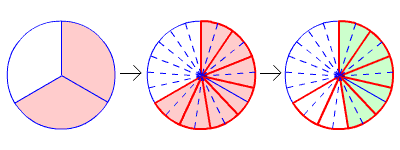# Multiplying a Fraction by a Fraction

To multiply two fractions, just multiply the numerators to get the numerator of the product, and multiply the denominators to get the denominator of the product.

$\frac{a}{b}\cdot \frac{c}{d}=\frac{a\text{\hspace{0.17em}}\cdot \text{\hspace{0.17em}}c}{b\text{\hspace{0.17em}}\cdot \text{\hspace{0.17em}}d}$

For example:

$\frac{2}{3}\cdot \frac{5}{7}=\frac{2\text{\hspace{0.17em}}\cdot \text{\hspace{0.17em}}5}{3\text{\hspace{0.17em}}\cdot \text{\hspace{0.17em}}7}$

$\text{\hspace{0.17em}}\text{\hspace{0.17em}}\text{\hspace{0.17em}}\text{\hspace{0.17em}}\text{\hspace{0.17em}}\text{\hspace{0.17em}}\text{\hspace{0.17em}}\text{\hspace{0.17em}}\text{\hspace{0.17em}}\text{\hspace{0.17em}}\text{\hspace{0.17em}}=\frac{10}{21}$

You can visualize this by starting with a drawing of $\frac{2}{3}$ , then finding $\frac{5}{7}$ of that quantity. Divide the $\frac{2}{3}$ piece into 7 pieces of equal size. Then shade 5 of them.Note that, even if the two fractions you're multiplying are both in simplest form , the result may not be:

$\frac{2}{5}\cdot \frac{3}{4}=\frac{2\text{\hspace{0.17em}}\cdot \text{\hspace{0.17em}}3}{5\text{\hspace{0.17em}}\cdot \text{\hspace{0.17em}}4}$

$\text{\hspace{0.17em}}\text{\hspace{0.17em}}\text{\hspace{0.17em}}\text{\hspace{0.17em}}\text{\hspace{0.17em}}\text{\hspace{0.17em}}\text{\hspace{0.17em}}\text{\hspace{0.17em}}\text{\hspace{0.17em}}\text{\hspace{0.17em}}=\frac{6}{20}$

This answer is correct, but it's better if you reduce the fraction (write it in simplest form). Factor out a 2 from both the numerator and denominator, and then cancel the common factor.

$=\frac{\overline{)2}\cdot \text{\hspace{0.17em}}3}{\overline{)2}\cdot \text{\hspace{0.17em}}10}$

$=\frac{3}{10}$

Note: If you're careful, you can speed up this process by reducing first , before multiplying.

$\frac{2}{5}\cdot \frac{3}{4}=\frac{\overline{)2}}{5}\cdot \frac{3}{2\text{\hspace{0.17em}}\cdot \overline{)2}}$

$=\frac{3}{5\text{\hspace{0.17em}}\cdot \text{\hspace{0.17em}}2}$

$=\frac{3}{10}$